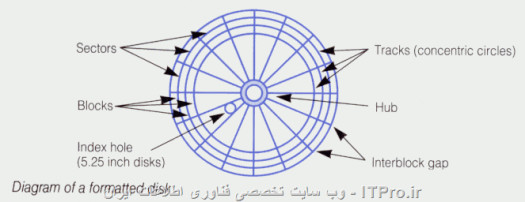# Area & ARC Lengths Assessment Test

Approved & Edited by ProProfs Editorial Team
At ProProfs Quizzes, our dedicated in-house team of experts takes pride in their work. With a sharp eye for detail, they meticulously review each quiz. This ensures that every quiz, taken by over 100 million users, meets our standards of accuracy, clarity, and engagement.
| Written by Cripstwick
C
Cripstwick
Community Contributor
Quizzes Created: 693 | Total Attempts: 678,987
Questions: 10 | Attempts: 400SettingsTake our assessment test to assess your knowledge of area and arc lengths using calculus, a mathematical topic with formulas and calculations. Challenge yourself!

• 1.

### The distance from the center to circumference of a circle is the...

• A.

Length

• B.

• C.

Arc

• D.

Diameter

Explanation
The distance from the center to the circumference of a circle is known as the radius. The radius is a line segment that connects the center of the circle to any point on its circumference. It is a fundamental measurement in geometry and is used to calculate various properties of a circle, such as its area and circumference.

Rate this question:

• 2.

### The central angle that subtends an arc length of one radius is...

• A.

• B.

• C.

2pi

• D.

Pi

Explanation
A central angle that subtends an arc length of one radius is defined as one radian. In a circle, the circumference is equal to 2π times the radius. Therefore, if the arc length is equal to the radius, it means that the angle formed by that arc is equal to one radian.

Rate this question:

• 3.

### The arc measure of the central angle of an entire circle is...

• A.

360

• B.

270

• C.

100

• D.

50

A. 360
Explanation
The arc measure of the central angle of an entire circle is 360 degrees. This is because a circle is defined as having 360 degrees in total. Therefore, the central angle, which spans the entire circle, will also have a measure of 360 degrees.

Rate this question:

• 4.

### The radian measure of the central angle of an entire circle is...

• A.

2pi

• B.

1pi

• C.

1

• D.

Pi

A. 2pi
Explanation
The radian measure of the central angle of an entire circle is 2pi because a full circle is divided into 360 degrees, and each degree is equal to pi/180 radians. Therefore, multiplying 360 degrees by pi/180 radians per degree gives us 2pi radians.

Rate this question:

• 5.

### Any portion, other than the entire curve of the circumference of a circle is the...

• A.

Arc

• B.

• C.

Point

• D.

Pole

A. Arc
Explanation
The correct answer is Arc. An arc is any portion of the circumference of a circle. It is a curved line that connects two points on the circumference of a circle. Unlike a full circle, an arc does not complete the entire circumference. It can be a small or large portion of the circle, depending on the points it connects.

Rate this question:

• 6.

### What is the circumference of a circle?

• A.

• B.

2pi

• C.

Pi

• D.

Explanation
The circumference of a circle is the distance around its outer edge. It can be calculated by multiplying the diameter of the circle by pi (π), which is approximately 3.14159. The given answer, 2×pi×radius, is the formula for finding the circumference of a circle using the radius. It is derived from the fact that the diameter of a circle is twice the radius. Therefore, multiplying the radius by 2 and then by pi gives us the circumference of the circle.

Rate this question:

• 7.

### How many degrees does a circle have?

• A.

90

• B.

360

• C.

270

• D.

400

B. 360
Explanation
A circle has 360 degrees because a circle is a complete revolution or a full rotation. Each degree represents a fraction of the entire circle, and since a circle is a closed shape with no angles, it has 360 degrees in total.

Rate this question:

• 8.

### What is the standard unit of angular measure?

• A.

• B.

Degree

• C.

Cm

• D.

Meters

Explanation
The standard unit of angular measure is the radian. It is used to measure angles in a circle, where one radian is equal to the angle subtended by an arc that is equal in length to the radius of the circle. The radian is widely used in mathematics and physics because it simplifies calculations involving angles and trigonometric functions. Degree, cm, and meters are not standard units of angular measure and are used to measure other quantities such as length.

Rate this question:

• 9.

### An arc of a circle having measure greater than or equal to radians is...

• A.

• B.

Major arc

• C.

Arc length

• D.

Diameter

B. Major arc
Explanation
A major arc is an arc of a circle that measures greater than or equal to radians. It is called a major arc because it covers a larger portion of the circumference compared to a minor arc. The measure of a major arc is equal to the measure of the central angle that intercepts it. Therefore, a major arc is the correct answer in this case.

Rate this question:

• 10.

### A straight line segment whose endpoints both lie on the circle is called...

• A.

Angle

• B.

Point

• C.

Dot

• D.

ChordBack to top